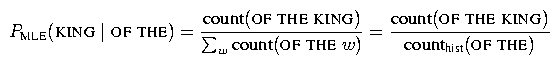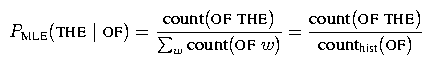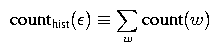# 2. Part 1: Implement n-gram counting

## 2.1. The Big Picture

For this lab, we will be compiling the code you write into the program EvalLMLab3. Here is an outline of what this program does:

• training phase

• reset all n-gram counts to 0

• for each sentence in the training data

• update n-gram counts (A)

• evaluation phase

• for each sentence to be evaluated

• for each n-gram in the sentence

• call smoothing routine to evaluate probability of n-gram given training counts (B)

• compute overall perplexity of evaluation data from n-gram probabilities

In the first part of the lab, you'll be writing the code that does step (A). In the next two parts of the lab, you'll be writing step (B) for two different smoothing algorithms.

## 2.2. This Part

In this part, you will be writing code to collect all of the n-gram counts needed in building a trigram model given some text. For example, consider trying to compute the probability of the word KING following the words OF THE. The maximum likelihood estimate of this trigram probability is:Thus, to compute this probability we need to collect the count of the trigram OF THE KING in the training data as well as the count of the bigram history OF THE. (The history is whatever words in the past we are conditioning on.) When building smoothed trigram LM's, we also need to compute bigram and unigram probabilities and thus also need to collect the relevant counts for these lower-order distributions.

Before we continue, let us clarify some terminology. Consider the maximum likelihood estimate for the bigram probability of the word THE following OF:Notice the term count(OF THE) in this equation and the term counthist(OF THE) in the last equation. We refer to the former count as a regular bigram count and the latter count as a bigram history count. While these two counts will be the same for most pairs of words, they won't be the same for all pairs and so we distinguish between the two. Specifically, the history count is used for normalization, and so is defined asA related point that is worth mentioning is that it is useful to have the concept of a 0-gram history. Just as we use unigram history counts in computing bigram probabilities, we use 0-gram history counts in computing unigram probabilities. We use the notation counthist(epsilon) to denote the 0-gram history count, and it is defined similarly as above, i.e.,In practice, instead of working directly with strings when collecting counts, all words are first converted to a unique integer index; e.g., the words OF, THE, and KING might be encoded as the integers 1, 2, and 3, respectively. In this lab, the words in the training data have been converted to integers for you. To see the mapping from words to integers, check out the file vocab.map in the directory ~stanchen/e6884/lab3/. As mentioned in lecture, in practice it is much easier to fix the set of words that the LM assigns (nonzero) probabilities to beforehand (rather than allowing any possible word spelling); this set of words is called the vocabulary. When encountering a word outside the vocabulary, one typically maps this word to a distinguished word, the unknown token, which we call <UNK> in this lab. The unknown token is treated like any other word in the vocabulary, and the probability assigned to predicting the unknown token (in some context) can be interpreted as the sum of the probabilities of predicting any word not in the vocabulary (in that context).

To prepare for the exercise, create the relevant subdirectory and copy over the needed files:
 ```mkdir -p ~/e6884/lab3/ cd ~/e6884/lab3/ cp ~stanchen/e6884/lab3/Lab3_LM.C . cp ~stanchen/e6884/lab3/.mk_chain . ```
Your job in this part is to fill in the sections between the markers BEGIN_LAB and END_LAB in the function `count_sentence()` in the file Lab3_LM.C. Read this file to see what input and output structures need to be accessed. This routine corresponds to step (A) in the pseudocode listed in Section 2.1. In this function, you will be passed a sentence (expressed as an array of integer word indices) and will need to update all relevant regular n-gram counts (trigram, bigram, and unigram) and all relevant history n-gram counts (bigram, unigram, and 0-gram). All of these counts will be initialized to zero for you.

In addition, for Witten-Bell smoothing (to be implemented in Part 3), you will also need to compute how many unique words follow each bigram/unigram/0-gram history. We refer to this as a “1+” count, since this is the number of words with one or more counts following a history.

It is a little tricky to figure out exactly which n-grams to count in a sentence, namely at the sentence begins and ends. For more details, refer to slide 39 (entitled “Technical Details: Sentence Begin and Ends”) in the week 5 language modeling slides. The trigram counts to update correspond one-to-one to the trigram probabilities used in computing the trigram probability of a sentence. Bigram history counts can be defined in terms of trigram counts using the equation described earlier. How to do counting for lower-order models is defined analogously.

## 2.3. Compiling and testing

Your code will be compiled into the program EvalLMLab3, which constructs an n-gram language model from training data and then uses this LM to evaluate the probability and perplexity of some test data. To compile this program with your code, type
 ```smk EvalLMLab3 ```
in the directory containing your source files (which must also contain the file .mk_chain).

To run this program (training on 100 Switchboard sentences and evaluating on 10 other sentences), run
 ```lab3p1a.sh ```
(This script can be found in ~stanchen/pub/exec/, which should be on your path from Lab 0.) This shell script starts the executable EvalLMLab3 located in the current directory with the appropriate flags. To start up EvalLMLab3 in the debugger, add the flag -debug to the above line. The “correct” output can be found in the file p1a.out in ~stanchen/e6884/lab3/; your output should match the correct output exactly. For each trigram being evaluated in the evaluation set, the program is set up to output all of the relevant training counts for that trigram. (The count following the label hist types is the “1+” count.) The final cross-entropy/perplexity output will be bogus, since the code for computing LM probabilities won't be filled in until later in the lab. To see the training text used by this script, check out the file minitrain.txt in the directory ~stanchen/e6884/lab3/.

The instructions in lab3.txt will ask you to run the script lab3p1b.sh, which does the same thing as lab3p1a.sh except on a different 10-sentence test set.